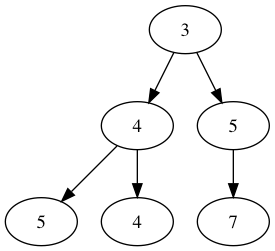# Introduction to Graphviz in Jupyter Notebook

## Goal¶

The goal in this post is to introduce graphviz to draw the graph when we explain graph-related algorithm e.g., tree, binary search etc. It would be nicer to have such a visualization to quickly digest problems and solutions.

Since we work with TreeNode and trees in a list-expresion e.g., [1, 2, null, 3] in LeetCode, the goal of this post is to easily convert the given tree in a list-expression into the visualization like below.

In :
from IPython.display import Image
Image('digraph.png')

Out:## Basic Usage¶

First of all, let's plot simplest two nodes and the edge between them.

In :
from graphviz import Digraph
# Create Digraph object
dot = Digraph()

In :
# Add nodes 1 and 2
dot.node('1')
dot.node('2')

# Add edge between 1 and 2
dot.edges(['12'])

In :
# Visualize the graph
dot

Out:

## Preperation of Tree Nodes¶

Now we look at an example of input tree nodes like below. We wrote the function to merge two trees in 617. Merge Two Binary Trees

In :
# Definition for a binary tree node.
class TreeNode(object):
def __init__(self, x):
self.val = x
self.left = None
self.right = None

class Solution(object):
def mergeTrees(self, t1, t2):
"""
:type t1: TreeNode
:type t2: TreeNode
:rtype: TreeNode
"""

if t1 and t2:
# Merge the values from two trees
node = TreeNode(t1.val + t2.val)

# Merge the left trees using the function recursively
node.left = self.mergeTrees(t1.left, t2.left)

# Merge the right trees using the function recursively
node.right = self.mergeTrees(t1.right, t2.right)

return node

else:
return t1 or t2


In :
# Example 1

# Left tree
t1 = TreeNode(1)
t1.left = TreeNode(3)
t1.left.left = TreeNode(5)
t1.right = TreeNode(2)

# Right tree
t2 = TreeNode(2)
t2.left = TreeNode(1)
t2.left.right = TreeNode(4)
t2.right = TreeNode(3)
t2.right.right = TreeNode(7)

In :
s = Solution()
merged_tree = s.mergeTrees(t1, t2)


Now we have three trees t1, t2, and merged_tree like below:

Tree 1 (t1)                    Tree 2 (t2)
1                         2
/ \                       / \
3   2                     1   3
/                           \   \
5                             4   7

Merged tree (merged_tree)
3
/ \
4   5
/ \   \
5   4   7

## Visualize Trees¶

### Manual creation¶

In :
# Create Digraph object
dot = Digraph()

# Add nodes
dot.node('1')
dot.node('3')
dot.node('2')
dot.node('5')

# Add edges
dot.edges(['12', '13', '35'])

# Visualize the graph
dot

Out:

### Programatic Creation¶

In :
def visualize_tree(tree):
def add_nodes_edges(tree, dot=None):
# Create Digraph object
if dot is None:
dot = Digraph()
dot.node(name=str(tree), label=str(tree.val))

# Add nodes
if tree.left:
dot.node(name=str(tree.left) ,label=str(tree.left.val))
dot.edge(str(tree), str(tree.left))
dot = add_nodes_edges(tree.left, dot=dot)

if tree.right:
dot.node(name=str(tree.right) ,label=str(tree.right.val))
dot.edge(str(tree), str(tree.right))
dot = add_nodes_edges(tree.right, dot=dot)

return dot

# Add nodes recursively and create a list of edges
dot = add_nodes_edges(tree)

# Visualize the graph
display(dot)

return dot


In :
dot = visualize_tree(t1)

In :
dot = visualize_tree(t2)

In :
dot = visualize_tree(merged_tree)

In :
# Save as svg file
dot.format = 'png'
dot.view(filename='digraph', directory='./')

Out:
'./digraph.png'

## Appendix¶

We need graphviz package. You can also refer to graphviz documentation

In :
!pip install graphviz

Requirement already satisfied: graphviz in /Users/212339410/anaconda3/envs/py367/lib/python3.6/site-packages (0.10.1)


## Comments

Comments powered by Disqus